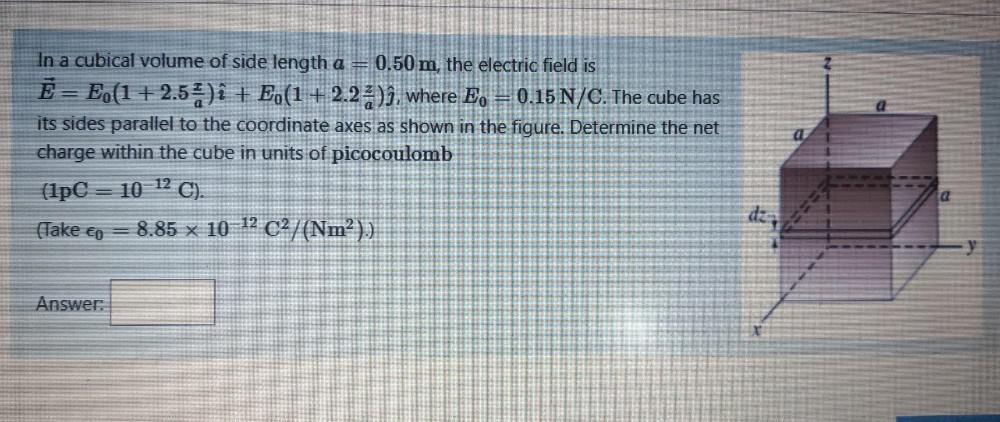Question:

# In a cubical volume of side length a = 0.50 m, the electric field is Ē= E,(1 + 2.54)i + E (1+2.23), where E, = 0.15 N/C. The cubIn a cubical volume of side length a = 0.50 m, the electric field is Ē= E,(1 + 2.54)i + E (1+2.23), where E, = 0.15 N/C. The cube has its sides parallel to the coordinate axes as shown in the figure. Determine the net charge within the cube in units of picocoulomb (1pC = 10 12 C). 8.85 x 10-12 C2/(Nm)) dz == (Take co Answer: